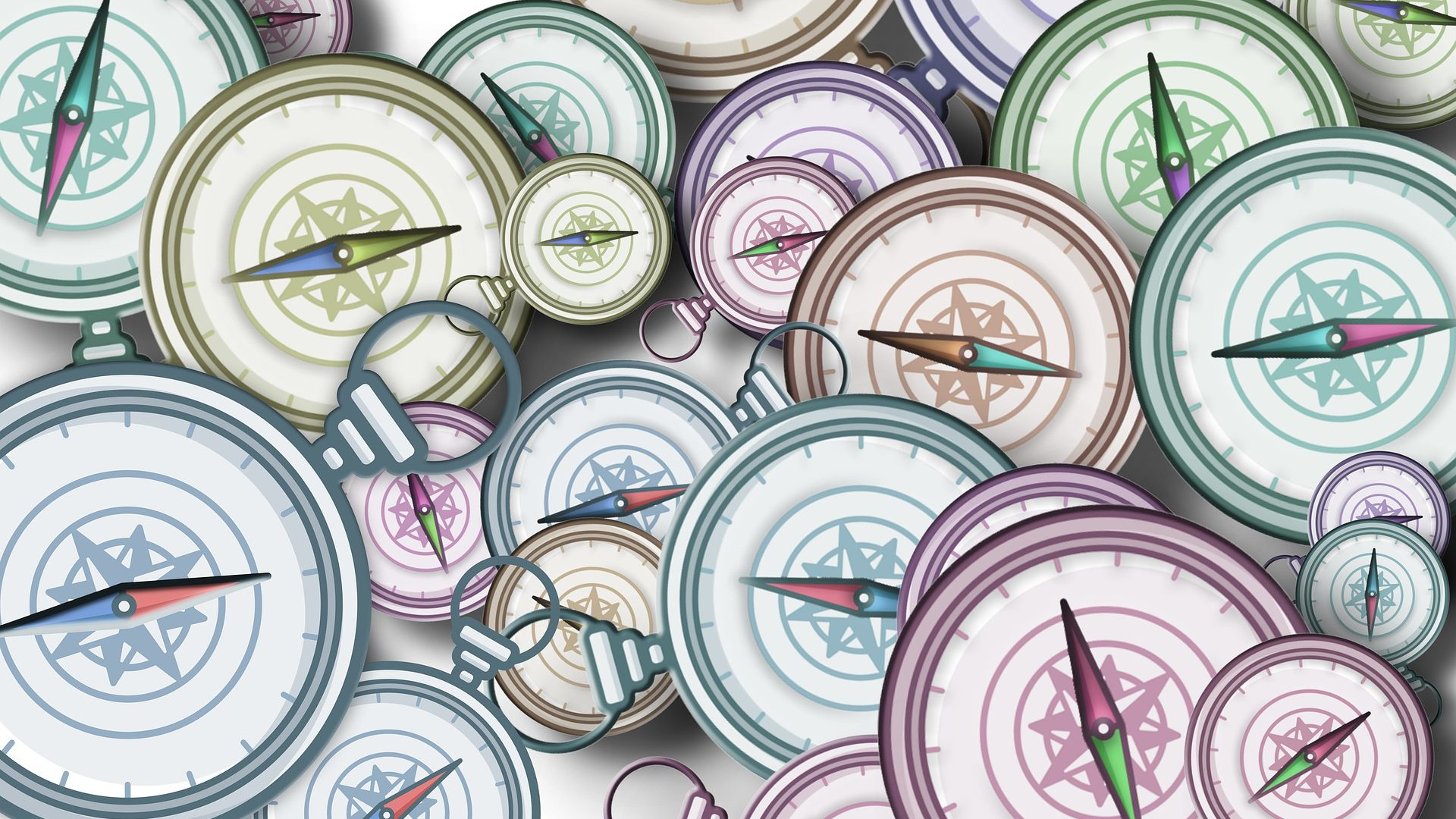# Deep Learning with Keras – Part 4: Classification

0
4180## Classification

### Introduction

Welcome to Part 3 of Deep Learning with Keras. The goal of this series is to get you familiar with the famous deep learning library Keras and how to use it for building various deep learning models. In this part we will focus on classification. Generally speaking, classification is the process of identifying to which predefined set of categories a new observation belongs.

Building a classification neural network requires some tweaks to what we have done before. Let us investigate the process next.

### Problem Definition

Consider a set of images containing handwritten digits from 0 to 9, our goal is to train a model that takes each picture and predict the correct digit it corresponds to. The data we are going to use is the famous MNIST database of handwritten digits. Keras already has this data available and we can load it as in the following:

``````from keras.datasets import mnist
(X_train, y_train), (X_test, y_test) = mnist.load_data()``````

Make sure you have an Internet connection the first time you run the above code since Keras will download the data from the web.

The obtained data is a set of 28*28 gray-scale images with 60000 training data and 10000 testing data. Each label is a number from 0-9 that represents the written digit in each image. The figure below shows a snapshot of the data:

### Data Preprocessing

As discussed in a previous article, we need to preprocess our image data. The following code reshapes and scales the images according to the techniques introduced here.

``````X_train_final = X_train.reshape(-1, 28*28) / 255.
X_test_final = X_test.reshape(-1, 28*28) / 255.``````

In addition to modifying images, we need to transform out labels to one-hot-encoded presentations in order to break the existing nominal relation. This is very simple in Keras and could be done as what follows:

``````from keras.utils import to_categorical
y_train_final = to_categorical(y_train)
y_test_final = to_categorical(y_test)``````

See the difference before and after encoding:

## Building the Network

Time to start building the network. We will build a simple one with one hidden layer and a special output layer that we will talk about soon. Let us start:

``````from keras import models, layers

model = models.Sequential()

model.add(layers.Dense(512, activation='relu', input_shape=(28*28, )))

model.add(layers.Dense(10, activation='softmax'))

model.compile('rmsprop', 'categorical_crossentropy', metrics=['acc'])``````

We have two new things here: 1) activation = ‘softmax’ and loss=’categorical_crossentropy’.

Softmax is a special activation function that transforms the output into probability values of each class. Therefore, with Dense(10) we will have 10 neurons each representing the probability of a given digit.

Categorical Cross-entropy is simply a loss function that calculates the error between the predicted digit and the actual one. We use categorical cross-entropy when we have 3 or more classes and binary cross-entropy when we have 2 classes.

### Training the Model

You should be familiar with the training process. We simply have to call the fit function passing our data:

``history = model.fit(X_train_final, y_train_final, epochs=10, batch_size=128, validation_split=0.2)``

Here we ran the model for 10 epochs with 20% validation data. The results are the following:

```Train on 48000 samples, validate on 12000 samples
Epoch 1/10 48000/48000 [==============================] - 5s 94us/step - loss: 0.2863 - acc: 0.9181 - val_loss: 0.1481 - val_acc: 0.9587
Epoch 2/10 48000/48000 [==============================] - 5s 102us/step - loss: 0.1184 - acc: 0.9654 - val_loss: 0.1040 - val_acc: 0.9702
Epoch 3/10 48000/48000 [==============================] - 5s 98us/step - loss: 0.0768 - acc: 0.9769 - val_loss: 0.0860 - val_acc: 0.9748
Epoch 4/10 48000/48000 [==============================] - 5s 100us/step - loss: 0.0548 - acc: 0.9836 - val_loss: 0.0963 - val_acc: 0.9713
Epoch 5/10 48000/48000 [==============================] - 5s 102us/step - loss: 0.0407 - acc: 0.9879 - val_loss: 0.0868 - val_acc: 0.9752
Epoch 6/10 48000/48000 [==============================] - 5s 114us/step - loss: 0.0303 - acc: 0.9911 - val_loss: 0.0875 - val_acc: 0.9763
Epoch 7/10 48000/48000 [==============================] - 5s 100us/step - loss: 0.0230 - acc: 0.9932 - val_loss: 0.0861 - val_acc: 0.9772
Epoch 8/10 48000/48000 [==============================] - 5s 102us/step - loss: 0.0179 - acc: 0.9948 - val_loss: 0.0844 - val_acc: 0.9778
Epoch 9/10 48000/48000 [==============================] - 5s 95us/step - loss: 0.0137 - acc: 0.9959 - val_loss: 0.0840 - val_acc: 0.9793
Epoch 10/10 48000/48000 [==============================] - 4s 88us/step - loss: 0.0101 - acc: 0.9973 - val_loss: 0.0879 - val_acc: 0.9797 ```

Impressive! We reached a 97% validation accuracy with a model as simple as this. Let us evaluate the model on the test set.

### Model Evaluation

``````model.evaluate(X_test_final, y_test_final)
# output
# [0.07102660850500979, 0.9799]``````

Very good results. 0.07 loss and again 97% accuracy.

### Final thoughts

In this article we covered classification and to implement it with Keras. We trained a model to predict handwritten digits and succeeded to reach a 97% accuracy. If you enjoyed the article please feel free to share it with your network. In case you have any questions do not hesitate to leave a comment below.

Note: This is a guest post, and opinion in this article is of the guest writer. If you have any issues with any of the articles posted at www.marktechpost.com please contact at [email protected]

This site uses Akismet to reduce spam. Learn how your comment data is processed.